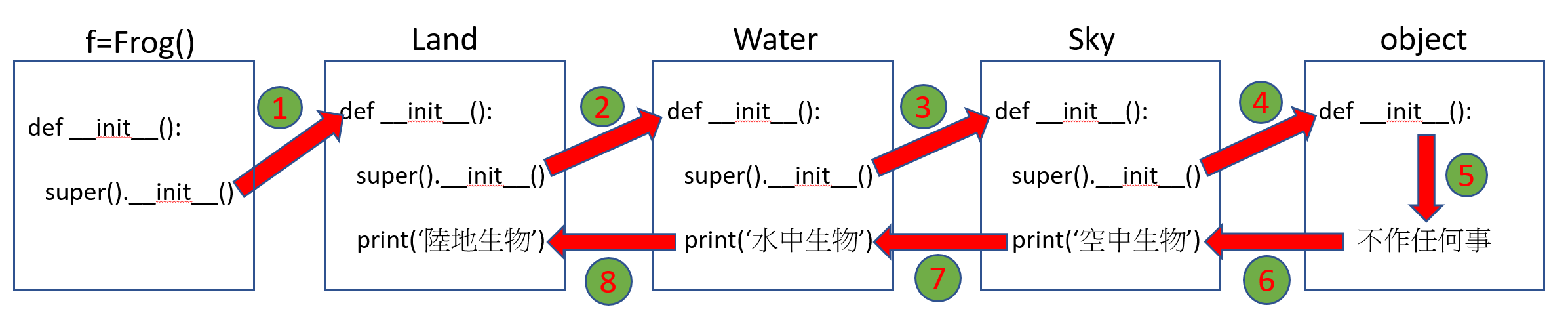# Python/Java/C#的不同

Python的子類別，可以繼承多個父類別，稱為多重繼承。但 Java，C# 只能使用單一繼承。

public class FirstApp(){    public static void main(String args){    }}class Land{}class Water{}class Sky{}class Frog extends Land{} <==只允許單一繼承Land

# 根本就沒有多重繼承

class Frog(Land, Water, Sky):    passif isinstance(Land, Water):    print("True")else:    print("False")結果 : False

1. 承接所有父類別的功能
2. 多型
3. 群組分類

# 承接所有父類別的功能

1. setLevel()
2. move()
3. breath()

class Pokemon(object):
def __init__(self, l=0):
self.level=l
def setLevel(self, l):
self.level=l
class Land(object):
def move(self):
return "Jumping....."
def breath(self):
return "Lung....."
class Water(object):
def move(self):
return "Swimming....."
class Sky(object):
def move(self):
return "Flying....."
class Frog(Pokemon, Land, Water):
pass
class Fish(Pokemon, Water):
pass
frog=Frog()
print("Frog move :  %s" % frog.move())
print("Frog breath :  %s" % frog.breath())

fish=Fish()
print("Fish move :  %s" % fish.move())

# 父類別相同名稱屬性及方法

Land及Water都具有相同的move()方法。 Land的move()方法是 Jumping, Water的 move()方法 是Swimming。

Frog繼承時，Land是寫在左邊，Water寫在右邊，所以是優先繼承了Land的move()。所以frog.move()就會執行Land的 move()，返回  “Jumping”。那有沒有可能使用 frog.move()去執行 Water 的 move() 並且返回 Swimming 呢? 答案是不行的，因為Water的 move() 被 Land 的 move() 覆蓋掉。

# mro

mro 全名為 Method Resolution Order(方法解析順序)，此函數記錄著繼承的順序。先看如下代碼的結果

import abc
class Move(abc.ABC):
@abc.abstractmethod
def move(self):
pass

class Land():
def __init__(self):
super().__init__()
print("陸地生物")
class Water():
def __init__(self):
super().__init__()
print("水中生物")
class Sky():
def __init__(self):
super().__init__()
print("空中生物")
class Frog(Land, Water, Sky):
def __init__(self):
super().__init__()
f=Frog()
print(Frog.mro())

[<class '__main__.Frog'>, <class '__main__.Land'>, <class '__main__.Water'>, <class '__main__.Sky'>, <class 'object'>]

Frog的下一個為Land, 然後依序為Water, Sky, object

# 執行所有父類別的建構子

class Land():
def __init__(self):
super().__init__()
print("陸地生物")
class Water():
def __init__(self):
super().__init__()
print("水中生物")
class Sky():
def __init__(self):
super().__init__()
print("空中生物")
class Frog(Land, Water, Sky):
def __init__(self):
super().__init__()
f=Frog()

1. 執行Frog的 __init__() ，其中的 super().__init__()是執行 Land的 __init__()
2. 在 Land的 __init()__又執行 super().__init__()，就是執行Water的 __init__()
3. 在Water的 __init__()又執行 super().__init__()，就是執行Sky的 __init__()
4. 在Sky的 __init__()又執行 super().__init__()，就是執行 object的 __init__()
5. 在 object的 __init__()，不執行任何東西，就直接返回。
6. 回到 Sky中，印出 “空中生物”
7. 回到 Water中，印出 “水中生物”
8. 回到 Land中，印出 “陸地生物”# 執行所有父類別的一般方法

from abc import abstractmethod
class Move():
@abstractmethod
def move(self):
pass
class Land(Move):
def __init__(self):
super().__init__()
print("陸地生物")
def move(self):
super().move()
print("用跑的")
class Water(Move):
def __init__(self):
super().__init__()
print("水中生物")
def move(self):
super().move()
print("用游的")
class Sky(Move):
def __init__(self):
super().__init__()
print("空中生物")
def move(self):
super().move()
print("用飛的")
class Frog(Land, Water, Sky):
def __init__(self):
super().__init__()
def move(self):
super().move()
f=Frog()
f.move()